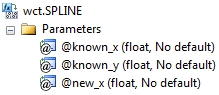# SQL Server cubic spline interpolation

SPLINE

Updated: 30 April 2011

Note: This documentation is for the SQL2008 (and later) version of this XLeratorDB function, it is not compatible with SQL Server 2005.

Use SPLINE to calculate the interpolated value of y given x in the array (x, y). SPLINE uses cubic spline interpolation. Unlike the scalar range query SPLINE function from earlier versions of XLeratorDB/math, the aggregate SPLINE function is not sensitive to the order of the array (x, y).
SyntaxArguments
@known_x
the x-values to be used in the interpolation calculation. @known_x must be of the type float or of a type that implicitly converts to float.
@known_y
the y-values to be used in the interpolation calculation. @known_y must be of the type float or of a type that implicitly converts to float.
@new_x
the new x-value for which you want INTERP to calculate the y-value.
Return Types
float
Remarks
·         For linear interpolation use the INTERP function.
·         For polynomial interpolation use the POLYINTERP function.
·         If an x-y pair contains a NULL, then the pair is not used in the interpolation calculation.
·         SPLINE will accept values for @new_x that are less than the minimum @known_x or greater than the maximum @known_x.
·         @new_x must remain invariant for the GROUP.
Examples
Given the following x- and y-values, we want to calculate the y-value for x = 0.75
SET NOCOUNT ON

SELECT wct.SPLINE(x,y,0.75) as SPLINE
FROM (VALUES
(0.5,0.479426),
(1,0.841471),
(1.5,0.997495),
(2,0.909297),
(2.5,0.598472),
(3,0.14112),
(3.5,-0.350783),
(4,-0.756802)
) n(x,y)

This produces the following result.
SPLINE
----------------------
0.675926077765373

In this example, we will interpolate using dates.
SET NOCOUNT ON

SELECT cast(x as datetime) as x,y
INTO #a
FROM (VALUES
('2012-Apr-30',0.0028),
('2013-Apr-30',0.0056),
('2014-Apr-30',0.0085),
('2016-Apr-30',0.0164),
('2018-Apr-30',0.0235),
('2021-Apr-30',0.0299),
('2031-Apr-30',0.0382),
('2041-Apr-30',0.0406)
) m(x, y)

SELECT wct.SPLINE(cast(x as float), y, cast(Cast('2012-Oct-31' as datetime) as float)) as SPLINE
from #a

DROP TABLE #a

This produces the following result.
SPLINE
----------------------
0.0042210614815398

We could have used the YEARFRAC function from XLeratorDB/financial, which makes the SQL somewhat simpler, but also permits interpolation using different interest bases. In this example, we will use YEARFRAC, using the 3-part function identifier because the YEARFRAC function is contained in a different database, to interpolate using the BOND interest basis.
SET NOCOUNT ON

SELECT cast(x as datetime) as x,y
INTO #a
FROM (VALUES
('2012-Apr-30',0.0028),
('2013-Apr-30',0.0056),
('2014-Apr-30',0.0085),
('2016-Apr-30',0.0164),
('2018-Apr-30',0.0235),
('2021-Apr-30',0.0299),
('2031-Apr-30',0.0382),
('2041-Apr-30',0.0406)
) m(x, y)

SELECT wct.SPLINE(
westclintech.wct.YEARFRAC('2011-Apr-30',x,0)
,y
,westclintech.wct.YEARFRAC('2011-Apr-30','2012-Oct-31',0)
) as SPLINE
FROM #a

DROP TABLE #a
This produces the following result.
SPLINE
----------------------
0.00420966880550746

In this example, we provide a @new_x value that is less than the minimum @known_x value.
SET NOCOUNT ON

SELECT wct.SPLINE(x, y, -3) as SPLINE
FROM (VALUES
(-2,-2),
(-1,-0.5),
(0,1),
(1,2.5),
(2,4),
(3,5.5)
) n(x, y)
This produces the following result.
SPLINE
----------------------
-3.5

In this example, we provide a @new_x value that is greater than the maximum @known_x value.
SET NOCOUNT ON

SELECT wct.SPLINE(x, y, 4) as SPLINE
FROM (VALUES
(-2,-2),
(-1,-0.5),
(0,1),
(1,2.5),
(2,4),
(3,5.5)
) n(x, y)
This produces the following result.
SPLINE
----------------------
7

In this example, one of the @known_y values is NULL.
SET NOCOUNT ON

SELECT wct.SPLINE(x, y, 1.5) as SPLINE
FROM (VALUES
(-2,-2),
(-1,-0.5),
(0,1),
(1,NULL),
(2,4),
(3,5.5)
) n(x, y)
This produces the following result.
SPLINE
----------------------
3.25

In this example, one of the @known_x values is NULL.
SET NOCOUNT ON

SELECT wct.SPLINE(x, y, 1.5) as SPLINE
FROM (VALUES
(-2,-2),
(-1,-0.5),
(0,1),
(1,2.5),
(NULL,4),
(3,5.5)
) n(x, y)
This produces the following result.
SPLINE
----------------------

3.25

### SupportCopyright 2008-2023 Westclintech LLC         Privacy Policy        Terms of Service